# The Multiplicative Inverse

The Multiplicative Inverse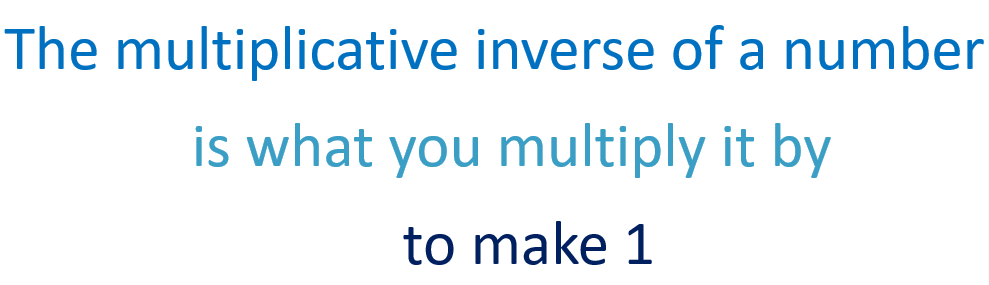• The multiplicative inverse of a number is what you multiply it by to make 1.
• For a fraction, the multiplicative inverse is the fraction flipped.
• This is called the reciprocal of the fraction.
• We can see that 3/5 × 5/3 = 15/15.
• 15/15 = 1 because 15 ÷ 15 = 1.• The multiplicative inverse of a whole number is the fraction of 1 over that number.
• For example, half of 2 is 1.
• The multiplicative inverse of 2 is 1/2.

The multiplicative inverse of a number is the number that you multiply it by to make an answer of 1.• The multilpicative inverse of 1/5 is 5.
• This is because 1/5 × 5 = 1.
• We know this since one fifth of 5 is 1.
• The multiplicative inverse of a unit fraction is simply the denominator.
• This means that if the fraction numerator is 1, the multiplicative inverse is the value of the denominator.Supporting Lessons# The Multiplicative Inverse

## What is the Multiplicative Inverse?

The multiplicative inverse of a number is what you multiply it by to make 1. For example, the multiplicative inverse of 2 is 1/2 because 2 × 1/2 = 1.Half of 2 is 1 and so, the multiplicative inverse of 2 is 1.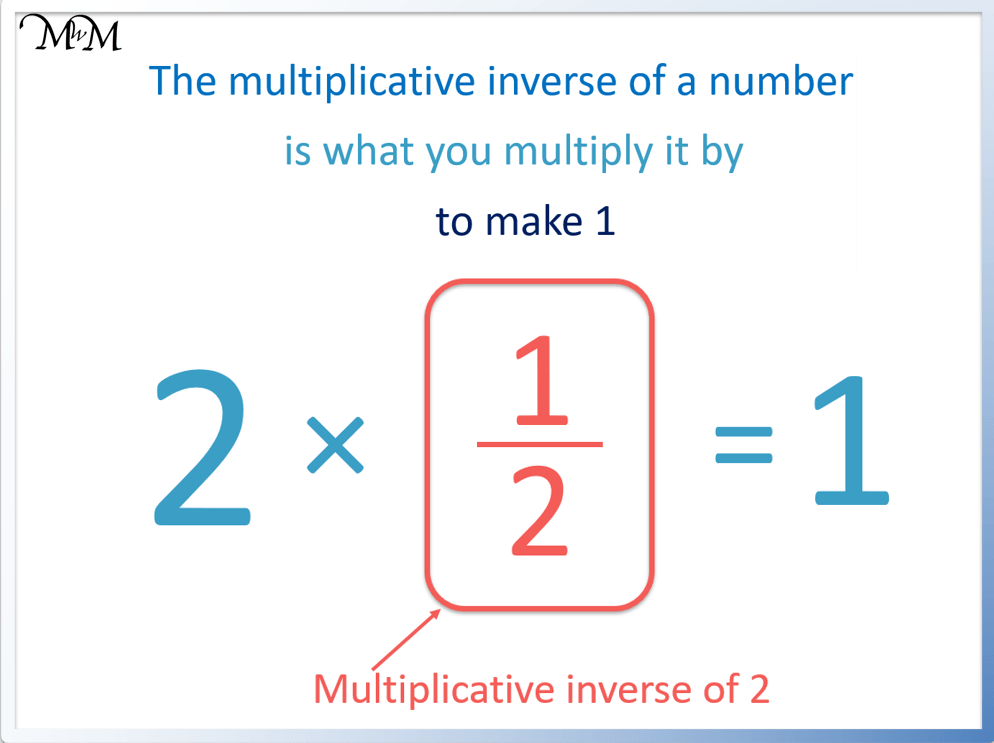## How to Find the Multiplicative Inverse

To find the multiplicative inverse of a number, write its reciprocal. For a fraction, this means switching the numerator and denominator. For a whole number, the multiplicative inverse is 1 over this number. For example, the multiplicative inverse of 2/3 is 3/2 and the multiplicative inverse of 3 is 1/3.

The multiplicative inverse of a number is the value that we multiply it by to make an answer of 1. When we multiply a number by its multiplicative inverse, the answer must be equal to 1.

To find the reciprocal of a fraction, switch the numerator on top with the denominator on the bottom. The fraction will be upside down.

For example, the reciprocal of 2/3 is 3/2.We can see that when we multiply these fractions, the answer is 1 and so, they are multiplicative inverses of each other.

Multipling the numerators we get 6 and multiplying the denominators we get 6.

6 out of 6 is one whole.

In this example, one third of 3 is 1 and so, 3 and 1/3 are multiplicative inverses of each other.Here are some examples of finding the multiplicative inverse.

Number Multiplicative Inverse
2 1/2
10 1/10
1/3 3
3/4 4/3
5/2 2/5
-4 1/4
1/6 -6
3/7 7/3
1 1
0 No Multiplicative Inverse

## How to Find the Multiplicative Inverse of a Fraction

The multiplicative inverse of a fraction is its reciprocal. This means that the numerator and denominator values are switched. The multiplicative inverse of the fraction a/b is b/a. For example, the multiplicative inverse of 5/7 is 7/5.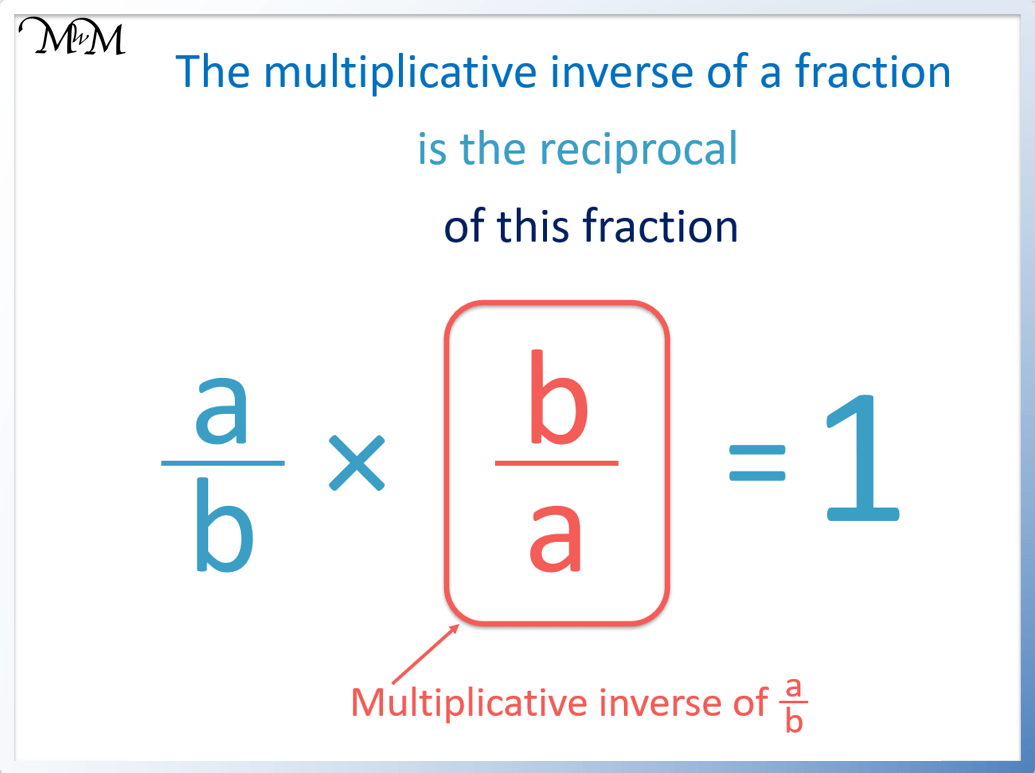We can see that we simply switch the numerator and denominator of a fraction to make the multiplicative inverse.It does not matter how large the numerator or denominator of a fraction is. The multiplicative inverse of the fraction is simply the reciprocal of the fraction. Switch the numerator and the denominator.

For example, the multiplicative inverse of 9/2 is 2/9.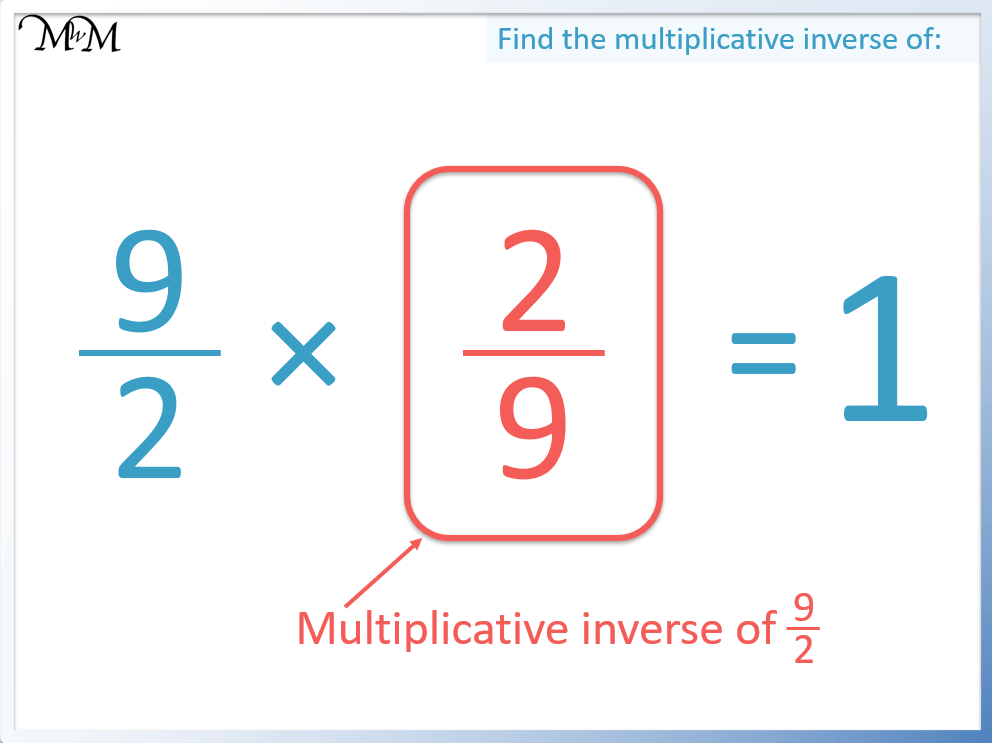The multiplicative inverse of a proper fraction is an improper fraction and the multiplicative inverse of an improper fraction is a proper fraction.

## Multiplicative Inverse of a Unit Fraction

The multiplicative inverse of a unit fraction is the denominator of the unit fraction. For any unit fraction 1/n, the multiplicative inverse is n. For example, the multiplicative inverse of 1/5 is 5 because 1/5 × 5 = 1.

A unit fraction must have a denominator of 1. The rule for finding the multiplicative inverse of a unit fraction is true for all unit fractions.We can see an example of finding the multiplicative inverse of a unit fraction below. One fifth of 5 is 1.## How to Find the Multiplicative Inverse of a Whole Number

The multiplicative inverse of a whole number is a unit fraction equal to 1 over that number. For any whole number ‘n’, the multiplicative inverse is 1/n. For example, the multiplicative inverse of 2 is 1/2 because 2 × 1/2 = 1.

This rule for the multiplicative inverse of a whole number works for any positive whole number value.The multiplicative inverse of 2 is 1/2 because one half of 2 is 1.To find the multiplicative inverse of a whole number, write a fraction with this number as the denominator and a numerator of 1.

For example, the multiplicative inverse of 3 is 1/3. The numerator is 1 and the denominator is 3.The multiplicative inverse of 7 is 1/7. This is because 7 × 1/7 = 1.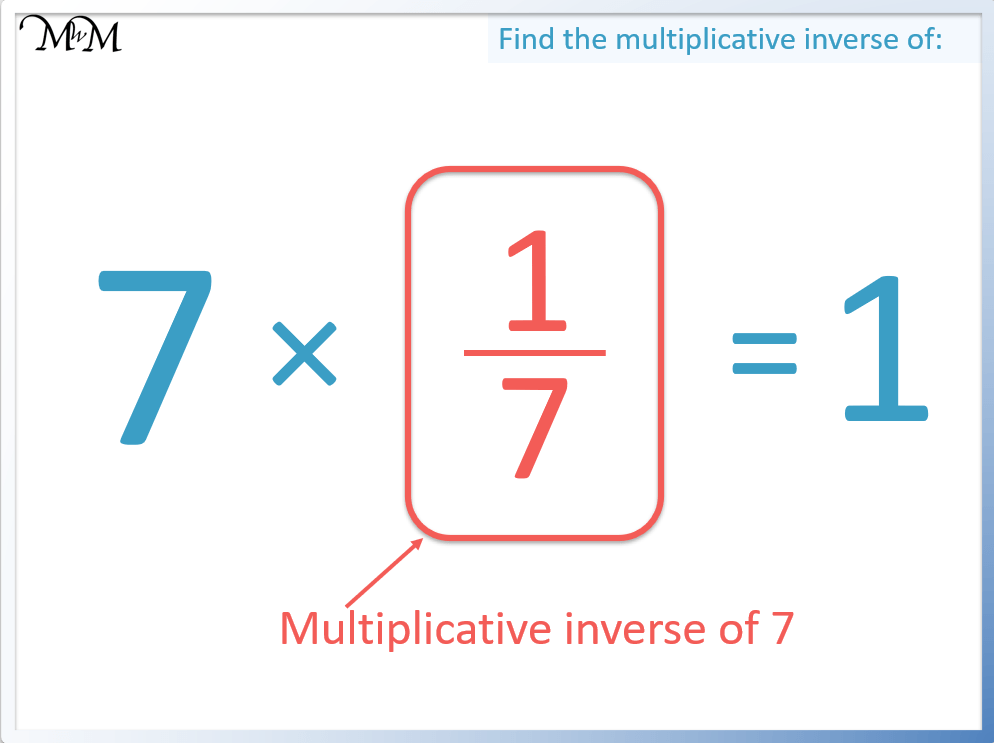The multiplicative inverse of 13 is 1/13. This is because 13 × 1/13 = 1.## Multiplicative Inverse of a Negative Number

The multiplicative inverse of a negative number is also negative. This is because the two negatives must cancel out when multiplied to make 1. For example, the multiplicative inverse of –2/5 is –5/2 because –2/5 × –5/2 = 1.

The multiplicative inverse of a number is the value we multiply it by to make an answer of 1.

For a fraction, the multiplicative inverse is simply the reciprical of the fraction. Below is an example of a negative fraction.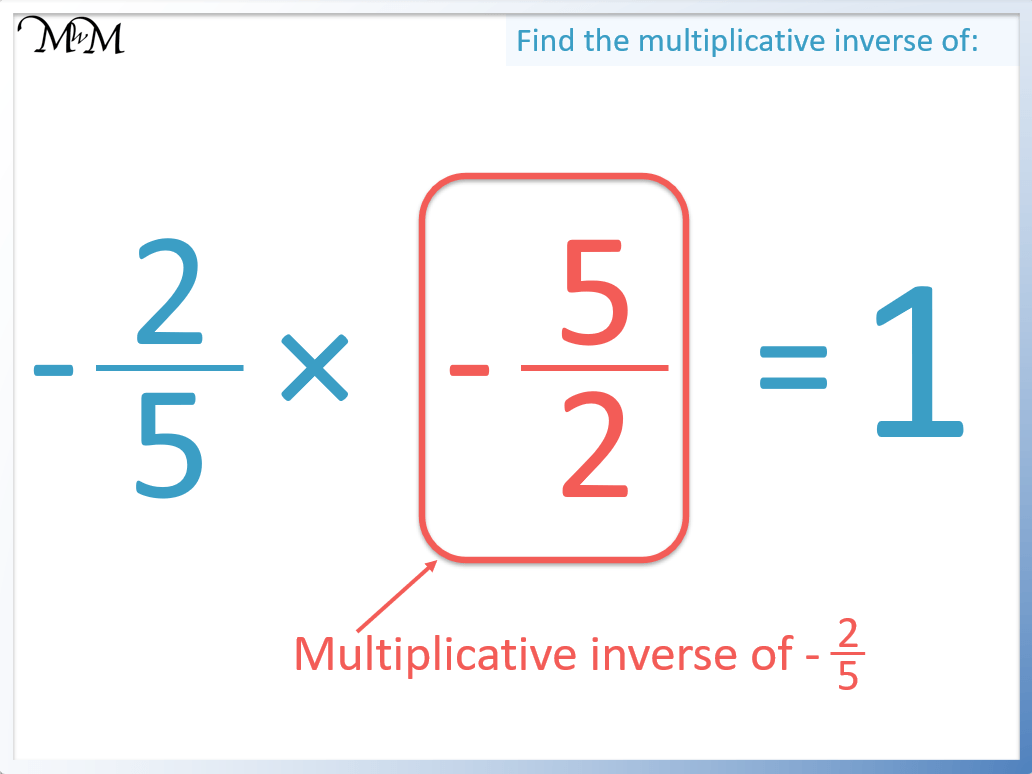We switch the numerator and denominator to find the reciprocal.

The multiplicative inverse of a negative number must also be negative. This is because two negatives multiplied together make a positive and when a number is multiplied by its multiplicative inverse, the answer must be positive 1.

Here is another example. We will find the multiplicative inverse of a negative whole number.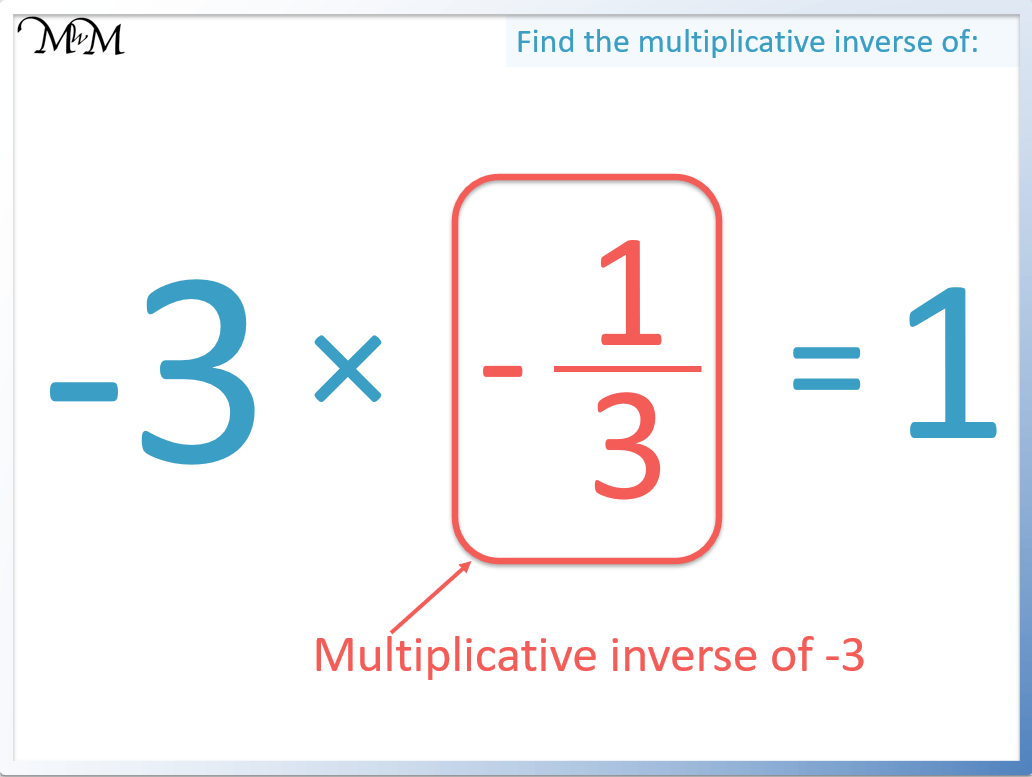The multiplicative inverse of 3 is 1/3. This is because one third of 3 is 1.

The multiplicative inverse of -3 is –1/3. This is because a negative number must be multiplied by another negative number to make a positive answer.

## What is the Multiplicative Inverse of 1?

The multiplicative inverse of a number is the value you multiply it by to make an answer of 1. Since 1 × 1 = 1, the multiplicative inverse of 1 is 1 itself.The only number that 1 can be multiplied by to make an answer of 1 is 1.

1 is the multiplicative inverse of 1.

1 can be written as a fraction as 1/1. To find the reciprocal of this fraction, we flip it so that the numerator becomes the denominator and vice versa.

The reciprocal of 1/1 is 1/1, which is the same as 1.

The reciprocal of 1 is 1.

## What is the Multiplicative Inverse of Zero?

There is no multiplicative inverse of zero. This is because there is no number that zero can be multiplied by to make 1. Zero is the only number that does not have a multiplicative inverse.Now try our lesson on Dividing Fractions by Whole Numbers where we learn how to divide a fraction by a whole number.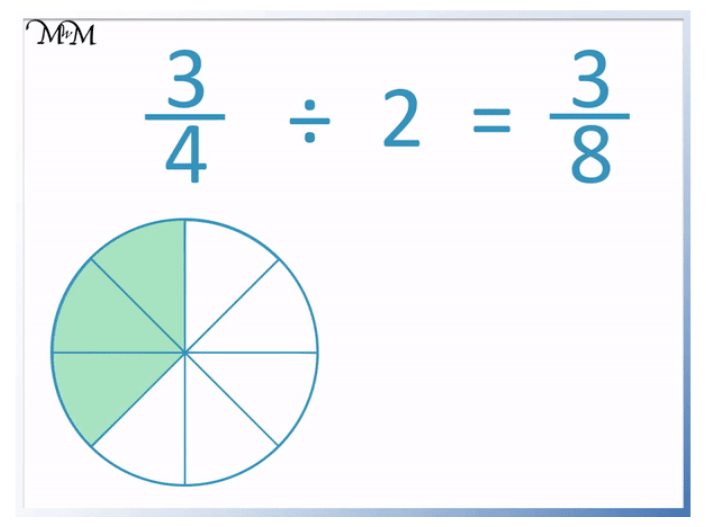error: Content is protected !!